Science, Maths & Technology

### Become an OU student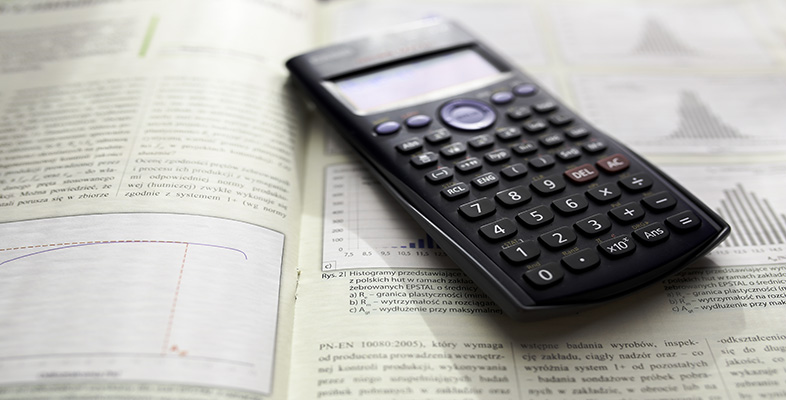Using a scientific calculator

Start this free course now. Just create an account and sign in. Enrol and complete the course for a free statement of participation or digital badge if available.

# 7 Trigonometric ratios on your calculator

There are various different units in which an angle can be measured, degrees being one of the possibilities. Before using your calculator to find the values of the trigonometric ratios of angles measured in degrees, you need to ensure that it is set to use the correct units.

Always check that your calculator is using the correct system of angle measurement before using trigonometric ratios.

Your calculator is set to use degrees if the display indicator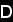is shown at the top of the screen. If you see the indicator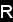or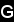, then your calculator is set to use different units for measuring angles.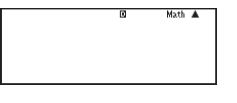Figure 9 The degrees setting

To set your calculator to work in degrees, use the key sequence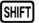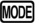(SETUP)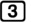(Deg).

To calculate the sine, cosine or tangent of an angle, press the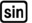,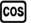or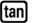key and then type in the size of the angle. Note that the,andkeys automatically open a bracket for you. If you are simply calculating the sine, cosine or tangent of an angle, just press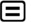after entering the angle – there is no need to close the bracket. If you are using these ratios as part of a larger calculation, then you will need to remember to close the bracket yourself (by pressing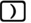) before entering the remainder of the calculation.

Some older models of calculator require the angle to be input first, followed by the,orbutton.

## Activity 16 Trigonometric ratios on your calculator

Calculate the value of each of the following using your calculator, giving your answers correct to 3 significant figures.

1.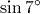2.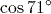3.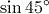4.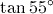5.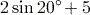6.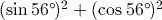1.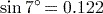(to 3 significant figures). If you got 0.657, then your calculator is currently set to calculate in radians, so reset it to work in degrees using(SETUP)(Deg).
2.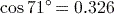(to 3 significant figures).
3.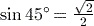or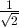or 0.707 (to 3 significant figures).
4.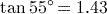(to 3 significant figures).
5.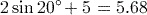(to 3 significant figures).

Remember to close the bracket that pressingopens before entering the ‘+5’. If you obtained the answer 0.845 (to 3 significant figures), you probably calculated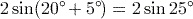instead.

Note that when entering this expression in your calculator, it is possible to omit explicitly entering the multiplication between the 2 and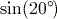since the calculator will assume it.

6.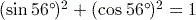.

Note that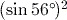means first find the sine of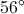, then square the answer. The key sequence to enterinto the calculator is thus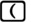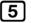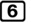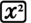. The firstis necessary to close the bracket automatically opened when pressing, and the second closes the bracket opened at the start of the sequence. Since the calculator evaluates the sine as soon as it encounters the first closing bracket, it is possible to enter this expression using the alternative sequence, but this is not recommended as the former is more clear.

It is a property of trigonometric ratios that for any angle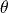,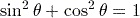.

You will notice from the answer to part (3) that the calculator displays the ratios of some angles as fractions, involving surds where needed, and not in decimal form.

The decimal form can be found usingor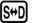.